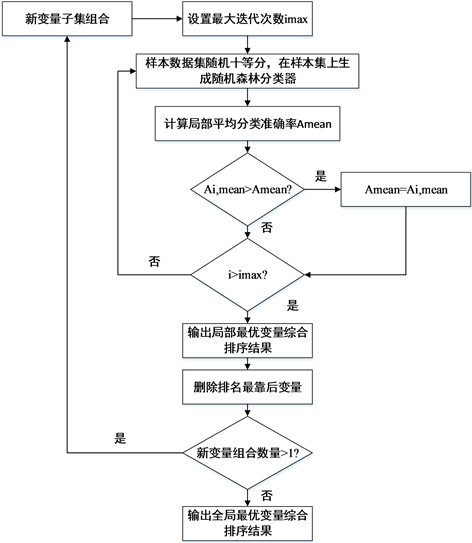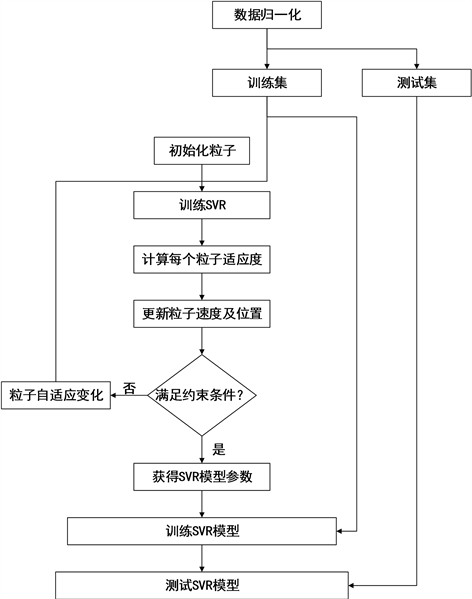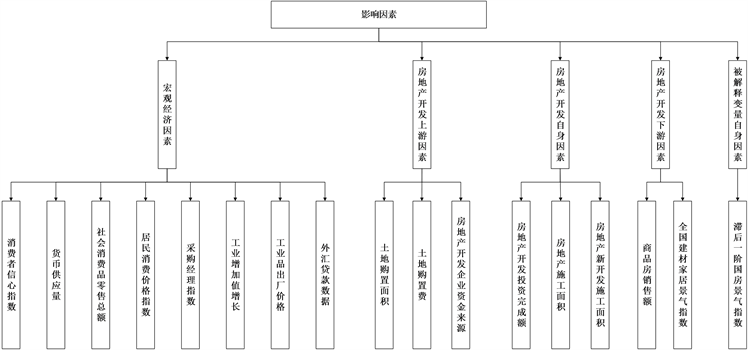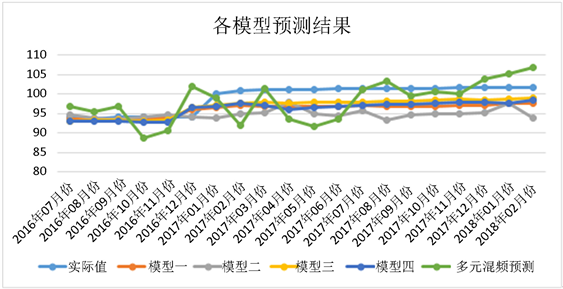# 基于混合核函数SVM修正MIDAS模型的房地产景气指数预测研究Study on Forecast of Real Estate Prosperity Index Based on MIDAS Model Corrected by Mixed Kernel Function SVM

DOI: 10.12677/AAM.2018.712182, PDF, HTML, XML, 下载: 478  浏览: 2,417

Abstract: The real estate market has the characteristics of multi influence factors and nonlinear fluctuations. With the further increase of the availability of relevant data information, the frequency difference and mutual interference between the factors cannot be ignored. Based on the existing research, we considered both the Baidu index with strong timeliness and related indicators of the real estate market with high relevance; we built the MIDAS model to solve the problem of frequency difference between Baidu index and real estate prosperity index, and established an optional packing and screening model random forests for the selection of features. Finally, based on the hybrid kernel SVR, we further modified the MIDAS prediction results. The empirical results showed that the model made full use of the mixed data information and also met the characteristics of the nonlinear fluctuations in the real estate market. The proposed model improved both the accuracy of forecasting and the volatility of the error.

1. 引言

Ghysels (2004)  等提出了混频数据抽样方法(MIDAS)，通过构建MIDAS模型可以实现对不同频率数据的处理，利用权重多项式对不同滞后期的变量系数进行描述和刻画解决了之前存在的“维数灾难”问题。Pan (2017)  等提出多输出混频支持向量机模型，利用低频数据实现对高频房地产及股票市场数据的预测，相较单输出SVM提升了模型的预测准确率。刘金全(2010)  基于MIDAS模型实证研究证明了混频宏观数据在经济应用中的有效性。

2. 模型构建

2.1. 模型基础

2.2. 模型步骤

1) 利用网络爬虫工具，根据定性分析以及百度推荐工具，获取影响房地产市场的相关百度指数数据指标，并基于AIC准则对相关指标进行逐步回归，选取最重要的前五个影响指标。

2) 选择从2011年1月至2018年1月的百度搜索数据以及国房景气指数作为研究对象，利用PSO-MIDAS单变量模型以及PSO-M-MIDAS多变量模型分别对百度指数与国房景气指数进行拟合，得到初始预测结果。

3) 选取房地产市场上下游的多个影响指标，构建指标体系，同时将国房景气指数进行趋势处理，分为上升趋势和下降趋势，构建随机森林为基础的封装特征选择模型，选取对房地产市场而言最为重要的影响因素作为输入变量。

4) 建立PSO-M-SVR模型，利用粒子群算法优化支持向量机相关参数，将混频抽样数据模型预测结果结合筛选指标作为输入变量，对初始预测结果进行修正，提高预测精度，并降低误差波动性。

2.3. PSO-M-MIDAS(m, k)模型

MIDAS模型区别于分布滞后多项模型的最重要性质，是其对于混频数据具有拟合预测能力，同时参数估计结果、模型预测精度均优于分布滞后模型。MIDAS模型在金融波动预测以及宏观经济预测等方面都有着广泛的应用，并取得了不错的预测效果。

Ghysels (2004)提出的MIDAS(m, k)模型包括参数化的多项式权重 $B\left({L}^{\frac{1}{m}};\theta \right)$ ，可以将高频数据 ${x}_{t}^{m}$ 与低频数据 ${y}_{t}$ 间建立回归联系：

${y}_{t}={\beta }_{0}+{\beta }_{1}B\left({L}^{\frac{1}{m}};\theta \right){x}_{t}^{m}+{\epsilon }_{t}$ (1)

${L}^{i/m}{x}_{t}^{\left(m\right)}={x}_{t-i/m}^{\left(m\right)},i=0,1,\cdots ,K-1,K$ 是高频数据的滞后阶数。常用的多项式权重函数包括Almon多项式函数，指数Almon多项式函数，β多项式函数，三种权重函数虽然表达方式不同，但都能够确保所使用的高频率滞后阶数的权重函数为正，同时多项式权重函数的表达式中实际包含了各项权重之和为1的前提条件。本文选择两参数的指数Almon多项式函数作为参数化权重函数，同时为了保证权重为正，方程误差小以及对应权重递减的特性，给定参数约束条件 ${\theta }_{1}\le 300,{\theta }_{2}\le 0$ 。两参数的指数Almon多项式函数的具体表达式为：

$w\left(k;\theta \right)=\frac{{\text{e}}^{\left({\theta }_{1}k+{\theta }_{2}k\right)}}{{\sum }_{k=1}^{K}\text{ }{\text{e}}^{\left({\theta }_{1}k+{\theta }_{2}k\right)}}$ (2)

Ghysels (2004)提出的多元混频预测模型M-MIDAS(m, K)基于单变量混频预测模型，任何一个经济系统都是一个复杂性系统，要想获得更为准确的预测效果需要利用多个解释变量的信息。M-MIDAS(m, K)模型的具体表达式为：

${y}_{t}={\beta }_{0}+{\sum }_{i=1}^{n}{\beta }_{i}B\left({L}^{\frac{1}{m}};\theta \right){x}_{i,t}^{m}+{\epsilon }_{t}$ (3)

2.4. 基于随机森林的封装特征选择

2.4.1. 基于随机森林的特征值重要性综合排序

$G{I}_{m}=1-{\sum }_{k=1}^{K}{p}_{mk}^{2}$ (4)

Gini指数的变化量表示了特征j在相关节点上的重要性，具体表达式为：

$VI{M}_{jm}^{gini}=G{I}_{m}-G{I}_{l}-G{I}_{r}$ (5)

$VI{M}_{ij}^{gini}={\sum }_{m\in M}VI{M}_{jm}^{gini}$ (6)

$VI{M}_{j}^{gini}={\sum }_{i=1}^{n}VI{M}_{ij}^{gini}$ (7)

2.4.2. 基于随机森林的封装特征选择

1) 设置迭代最大次数 ${i}_{\mathrm{max}}$

2) 给定需要排序的特征变量组合，将数据集随机分为十等份。

3) 在处理完成的数据集上生成随机森林训练模型，初步进行模型训练，在测试集上得到初始局部分类准确率 ${A}_{i}=A\left[1:10\right]$ ，初始局部平均分类准确率 ${A}_{mean}={\sum }_{i=1}^{10}{A}_{i}/10$

4) 进行迭代运算，在测试集上得到每次的局部分类准确率 ${A}_{i}$ ，并对 ${A}_{mean}$ 进行迭代更新，若 ${A}_{i-1,mean}\le {A}_{i,mean}$ ，则 ${A}_{mean}={A}_{i,mean}$

5) 若 $i>{i}_{\mathrm{max}}$ ，则迭代结束，否则返回第二步。

6) 得到同一组变量组合的最优平均预测准确度，并得到该组变量集合的特征综合重要性排序结果。因为是采用10折交叉验证，因此，特征j的重要性值为 ${B}_{j}={\sum }_{i=1}^{10}{S}_{ji}$${S}_{ji}$ 表示第j个特征在交叉验证第i组数据中的重要性值，进行降序排列。

7) 根据序列后向搜索方法，删除排名最低的特征值，形成的特征变量组合，并判断特征变量组合中的变量个数是否大于1，若大于1，则返回第一步。

8) 得到全局最优的分类准确率 ${A}_{global}$ ，返回其对应的特征重要性排序结果。

2.5. 粒子群优化多核支持向量机预测模型

${K}_{mix}=\theta {K}_{poly}+\left(1-\theta \right){K}_{k}$ (8)Figure 1. Package filtering based on random forest

PSO-M-SVR具体算法流程图如图2所示。

3. 实证分析

3.1. 数据选取及变量说明

3.1.1. 被解释变量

3.1.2. 混频预测模型解释变量Figure 2. Algorithm flowchart of PSO-M-SVR

3.1.3. 粒子群优化多核支持向量机模型解释变量

3.2. 结果分析

3.2.1. 混频数据预测模型结果分析Figure 3. Impact indicators of real estate market

$\text{MAPE}={\sum }_{i=1}^{n}\left(\frac{|\text{Prevalue}-\text{Actvalue}|}{\text{Actvalue}}\right)/n$ (9)

$\text{RMSE}={\sum }_{i=1}^{n}{\left(\text{Prevalue}-\text{Actvalue}\right)}^{2}/n$ (10)Table 1. Prediction results of multivariate and univariate MIDAS models

3.2.2. 基于随机森林的特征选择结果

3.2.3. 基于粒子群优化多核支持向量机模型预测结果分析Figure 4. Prediction results of different models

$\text{AE}=|\text{Prevalue}-\text{Actvalue}|/\text{Actvalue}$ (11)

4. 结论与展望Table 2. Prediction results of optimization and comparison models

  章伟. 粗糙集BP神经网络在房地产价格预测中的应用[J]. 计算机仿真, 2011, 28(7): 365-368.  刘彩云, 姚俭. 基于多因素影响的房地产价格预测模型[J]. 统计与决策, 2017(17): 33-38.  Del Giudice, V.D., Paola, P.D. and Forte, F. (2017) Using Genetic Algorithms for Real Estate Appraisals. Buildings, 2.  Bae, S.W., et al. (2018) Pre-dicting the Real Estate Price Index Using Machine Learning Methods and Time Series Analysis Model. Housing Studies, 26, 107-133.  Ginsberg, J., Mohebbi, M.H., Patel, R.S., et al. (2009) Detecting Influenza Epidemics Using Search Engine Query Data. Nature, 457, 1012-1014. https://doi.org/10.1038/nature07634  Askitas, N. and Zimmermann, K.F. (2009) Google Econometrics and Unemployment Forecasting. Discussion Papers of Diw Berlin, 55, 107-120.  董倩, 孙娜娜, 李伟. 基于网络搜索数据的房地产价格预测[J]. 统计研究, 2014, 31(10): 81-88.  孟雪井, 孟祥兰, 胡杨洋. 基于文本挖掘和百度指数的投资者情绪指数研究[J]. 宏观经济研究, 2016(1): 144-153.  Ghysels, E., Santa-Clara, P. and Valkanov, R. (2004) The MIDAS Touch: Mixed Data Sampling Re-gressions. Cirano Working Papers, 5, 512-517.  Pan, Y., Xiao, Z., Wang, X., et al. (2017) A Multiple Support Vector Machine Approach to Stock Index Forecasting with Mixed Frequency Sampling. Knowledge-Based Systems, 122, 90-102. https://doi.org/10.1016/j.knosys.2017.01.033  刘金全, 刘汉, 印重. 中国宏观经济混频数据模型应用——基于MIDAS模型的实证研究[J]. 经济科学, 2010, 32(5): 23-34.  李小琳, 孙玥, 刘洋. 基于SVM修正的模糊时间序列模型在沪指预测中的应用[J]. 中国科学技术大学学报, 2016(3): 238-246.  刘汉, 刘金全. 中国宏观经济总量的实时预报与短期预测——基于混频数据预测模型的实证研究[J]. 经济研究, 2011(3): 4-17.  蒋翠清, 王睿雅, 丁勇. 融入软信息的P2P网络借贷违约预测方法[J]. 中国管理科学, 2017(11): 12-21.  孙菲艳, 田雨波, 任作琳. 采用混合核支持向量机的DOA估计[J]. 电讯技术, 2016, 56(3): 302-307.